# Base stat application formula

#### Jediment

Just curious, is the formula for the application of a base stat known? I'm trying to figure it out exactly (and having some difficulty) so I just wanted to make sure my efforts aren't all for naught. It seems from what I've seen that in bx=s (b is the base stat and s is the stat without EVs at level 100), b and x are inversely proportional (though I'm currently trying to figure out how to expand on that, as I'm not the best mathematician in the world...)

#### ICanBeYourHero

Stat=2*Base+IV+(EVs/4)+5

Multiply it by 1.1 or 0.9 depending on nature.

#### Jediment

So then if EVs aren't applied (pokemon has 0 EVs) you can eliminate them from the formula I'm assuming. Thanks much for letting me know so I didn't waste any more time.

#### X-Act

##### np: Biffy Clyro - Shock Shock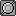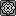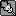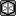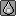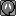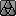I don't know from where you got those formulae Obi, but the HP one is wrong for sure (I tried it).

The correct formula is (correct for ALL stats, including HP):

Stat = (((2 * Base + IV + (EV / 4)) * Level / 100) + Num) * Mod

where

Base is your base stat
IV is the IV of that stat
EV is the EV of that stat
Level is your level
Mod is 1.1 if stat has beneficial nature, 0.9 if stat has hindering nature, 1 otherwise
Num is Level+10 if stat is HP, 5 otherwise

Make sure you round down the number you get after every calculation that gives you a decimal.

#### david stone

##### Fast-moving, smart, sexy and alarming.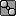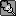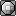Hmm actually if I had thought before posting, I would have realized the rounding things I mentioned are wrong. I'll edit my post to avoid confusion.

I didn't get the formula from any specific place, I just reverse-engineered it from Pokemon stats. Guess I wasn't careful enough.

#### scorchedsky

Are base stats just discovered through game code? Because I'd hate to think every one of those stats had to be reverse-engineered for every pokemon...

#### X-Act

##### np: Biffy Clyro - Shock ShockBase stats are extracted from the game code, yes.

#### Bourbon

I figure it out like this:

Base Stat * 2 + 5 + 63 (EVs) + 31 (IVs)

So for example:

100 * 2 = 200 + 99 is 299. Add 29 to get 328, do not times by 1.1... add the first two digits.